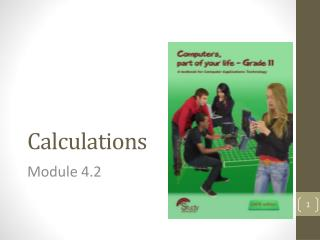DownloadDownload PresentationCalculations

# Calculations

Download Presentation## Calculations

- - - - - - - - - - - - - - - - - - - - - - - - - - - E N D - - - - - - - - - - - - - - - - - - - - - - - - - - -
##### Presentation Transcript

1. Calculations Module 4.2

2. Contents • Functions • Interpretation of error indicators • Test and improve your knowledge

3. Functions

4. The ROUND function • . The ROUND function can be used to round numbers to a specified number of decimal places after the nearest decimal point.

5. ROUND function vs. Increase/Decrease decimal However, the values stored in the cells, and which are used for calculations, will remain the same (even if they are displayed differently).

6. The LARGE and SMALL functions

7. Functions which can count (1)

8. Functions which can count (2) Note the use of quotation marks in the COUNTIF function.

9. Note: • The COUNTIF function is used to count the number of grade 10 learners, which is the number of times the value entered in B12 is found in the range C3:C10. • When we copy this function down, the row numbers of the range tested must not change. This is why we use absolute referencing here.

10. Other handy functions

11. Calculate the sum of certain cells = SUMIF(range, criteria, sum_range) • Range is the range of cells e.g. A2 to A12 (A2:A12) that is tested to determine if any cells in it meet the criteria. • Criteria determines which cells are added and may be numbers, text or an expression. • Sum_range are the actual cells that are added, if the corresponding cells in the range meet the criteria. Gebied is die deel bv. A2 tot A12 (A2:A12) wat getoets word of dit aan die kriteria voldoen. Kriteria bepaal watter selle bymekaar getel word en kan getalle, teks of ’n uitdrukking wees. Som_gebied is die selle wat bymekaar getel word indien die ooreenstemmende selle aan die kriteria voldoen.

12. Calculate the sum of certain cells Example = SUMIF(B2:B6, 11, C2:C6) • Tests the rangeB2:B6 • Criteria: the cell must contain the value 11. • If the value of the corresponding cell in column B is 11, it must be added. The result of the SUMIF function in cell C8 is R45.00 The cells that meet the criteria are B2, B4 and B5. The values in the corresponding cells in column C are R20.00 (C2), R10.00 (C4) and R15.00 (C5), which add up to R45.00. Gebied is die deel bv. A2 tot A12 (A2:A12) wat getoets word of dit aan die kriteria voldoen. Kriteria bepaal watter selle bymekaar getel word en kan getalle, teks of ’n uitdrukking wees. Som_gebied is die selle wat bymekaar getel word indien die ooreenstemmende selle aan die kriteria voldoen.

13. TIP Entering a SUMIF function is made easy using the Insert Function command on the Formulas tab.

14. Interpretation of error indicators

15. An error indicator always begins with a hash sign (‘#’)

16. Test and improve your knowledge

17. Overview of module

18. Important terms and concepts

19. Tips and recommendations • Always remember that the value displayed in a cell is not necessarily the same as what is stored in the cell • The ^ operator can be used instead of the POWER function - =POWER(5,3) and =5^3 will return exactly the same answer. • Use of absolute cell references for specifying the ranges in COUNTIF and SUMIF functions is more efficient than using relative cell references. =COUNTIF(range, criterion) = SUMIF(range, criteria, sum_range)